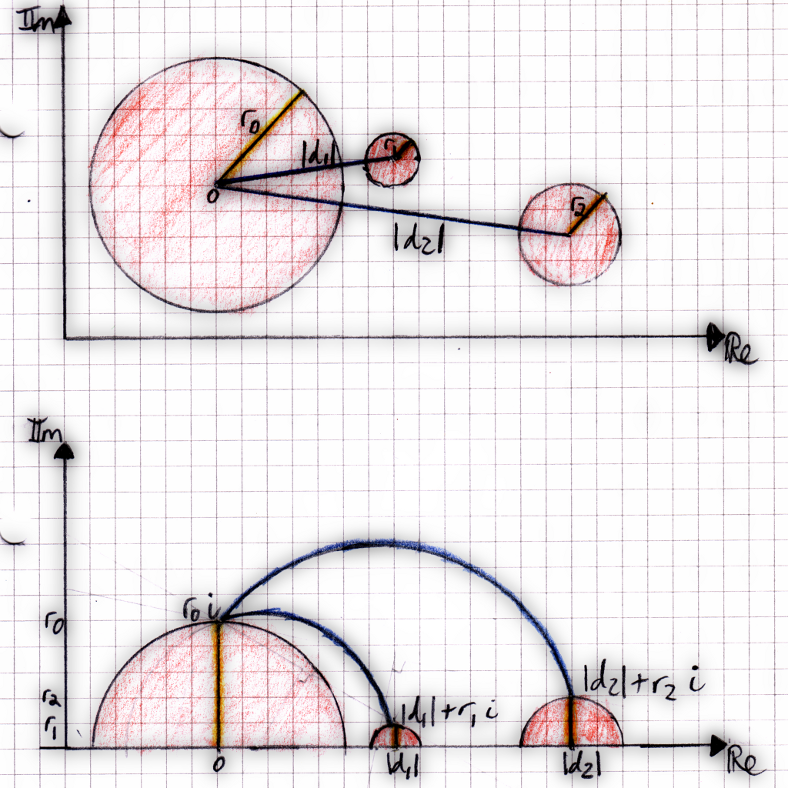# mathr / blog / #

## Poincaré half-plane metric for zoom animationThe diagram above shows two views: the top half is a view of the complex plane with some circles indicating viewports at different sizes (zoom levels) and locations. The problem I wanted was to solve was:

how might one derive the shortest animation path from one viewport to another.

My intuition was that the shortest path would involve zooming out, moving sideways, and zooming in again, but sharp corners between the zooming sections and the translating sections would be ugly, and almost certainly add to the length of the path. I remembered the diagrams on the wikipedia page on the Poincaré half-plane model of hyperbolic geometry, and thought that perhaps it would apply. In that model, the shortest path (aka a straight line) between two points in hyperbolic space maps to a circular arc through Euclidean space.

Mapping the radius of the view to the imaginary coordinate (height) in the half-plane, and the distance along the line between the centres of the viewports to the real coordinate, then the Poincaré half-plane geodesics (shortest paths) indeed zoom out, translate, and zoom in again in the smoothest possible way. This is shown in the bottom half of the diagram on this page.

(At this point I might remark that I tried implementing this some time ago in Mandulia, but I didn't understand it enough, I got as far as circular arcs but the scaling was all wrong and I had to apply many ad-hoc hacks to get it to look ok.)

Some experimentation followed, implemented like this in Haskell:

-- representation of viewports
data Poincare a = Poincare !(Complex a) !a deriving Show

-- hyperbolic distance between viewports
distance (Poincare x1 y1) (Poincare x2 y2) = acosh (1 + (magnitudeSquared dx + dy * dy) / (2 * y1 * y2))
where dx = x2 - x1 ; dy = y2 - y1

-- hyperbolic geodesic
-- the parameter t in [0..1] interpolates between the endpoints
geodesic p1@(Poincare x1 y1) p2@(Poincare x2 y2)
| x1 == x2  = \t -> Poincare x1 (y1 * (y2 / y1) ** t)
| otherwise = \t -> let x:+y = go (exp (t * dt)) in Poincare (x1 + (x :+ 0) * dir) y
where
go t = (b :+ (a * t)) / (d :+ (c * t))   -- interpolate geodesic
a =  d * y1
b = -c * y1
[c, d] = toList . nullVector \$ (2><2)[ eT * mdx, y2 - y1 * eT, y1 - eT * y2, mdx ]
eT = exp dt
dt = distance p1 p2    -- hyperbolic distance between viewports
dx = x2 - x1           -- vector between viewport centers
mdx = magnitude dx     -- distance between viewport centers
dir = cis (phase dx)   -- unit vector between viewport centers

The complicated bit is the computation of the geodesic parameters, which I derived like this:

      { start with a pair of equations for the end points }
(a i     + b) / (c i     + d) =     0     + i y1
(a i e^T + b) / (c i e^T + d) = |x2 - x1| + i y2
=>    { multiply out }
(a i     + b) = (c i     + d) * (    0     + i y1)
(a i e^T + b) = (c i e^T + d) * (|x2 - x1| + i y2)
=>    { equate real and imaginary parts of each equation }
a     =  d * y1
b     = -c * y1
a e^T = c e^T |x2 - x1| + d y2
b     = d     |x2 - x1| - c e^T y2
=>    { substitute a and b to get two equations in two variables }
d y1 e^T = c e^T |x2 - x1| + d y2
-c y1    = d     |x2 - x1| - c e^T y2
=>    { rearrange }
c e^T |x2 - x1|    + d (y2 - y1 e^T) = 0
c (-(e^T y2 - y1)) + d |x2 - x1|     = 0
=>    { express in matrix form }
M (c;d) = 0

Now, my first attempt failed miserably, because the standard methods to solve matrix equations see M (c;d) = 0 and end up finding the solution c = 0, d = 0, which makes a = 0 and b = 0, but this is useless as you end up with 0/0 which is undefined nonsense. Recalling linear algebra, I looked up the term null space and it indeed turned out to be what was needed here, namely to find a vector v such that M v = 0 and v /= 0. Handily there is an implementation in the hmatrix package, and as I only needed one such vector, the nullVector function was a perfect fit.

I made a demo of this technique in action (18MB), using ruff to find locations and gruff to render the animation.

References:

metric
Poincaré half-plane model metric (wiki)
geodesic
Poincaré half-plane model geodesic (wiki)
null space
Kernel matrix null space (wiki)